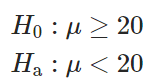# Consider the following hypothesis test:

Consider the following hypothesis test:A sample of 50 provided a sample mean of 19.4. The population standard deviation is 2.

a. Compute the value of the test statistic.
b. What is the p-value?
c. Using α = .05, what is your conclusion?
d. What is the rejection rule using the critical value? What is your conclusion?